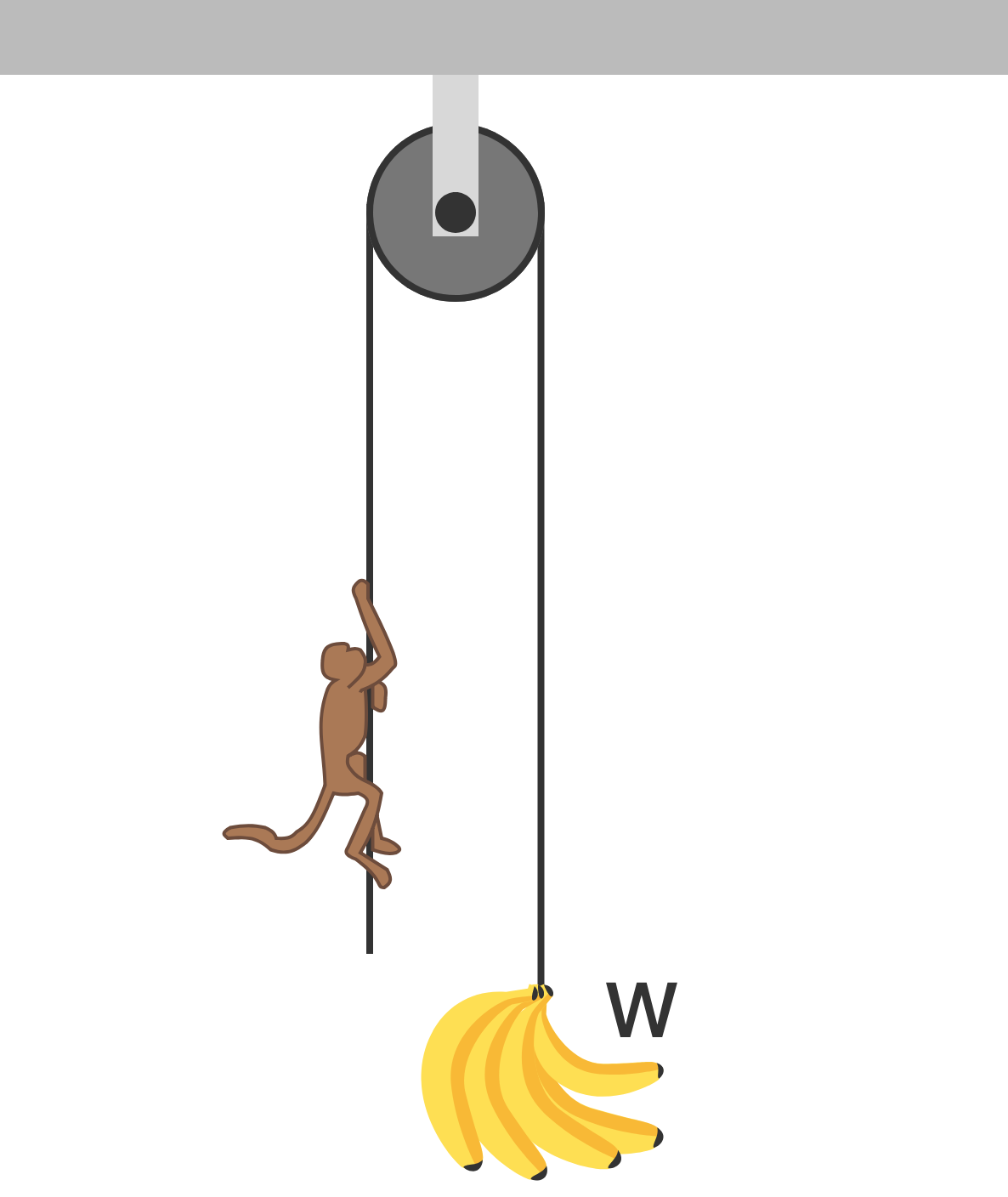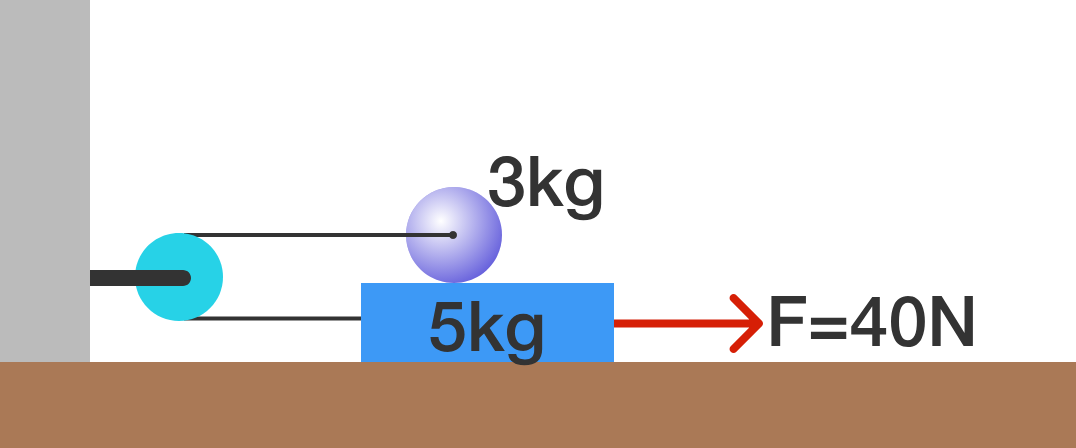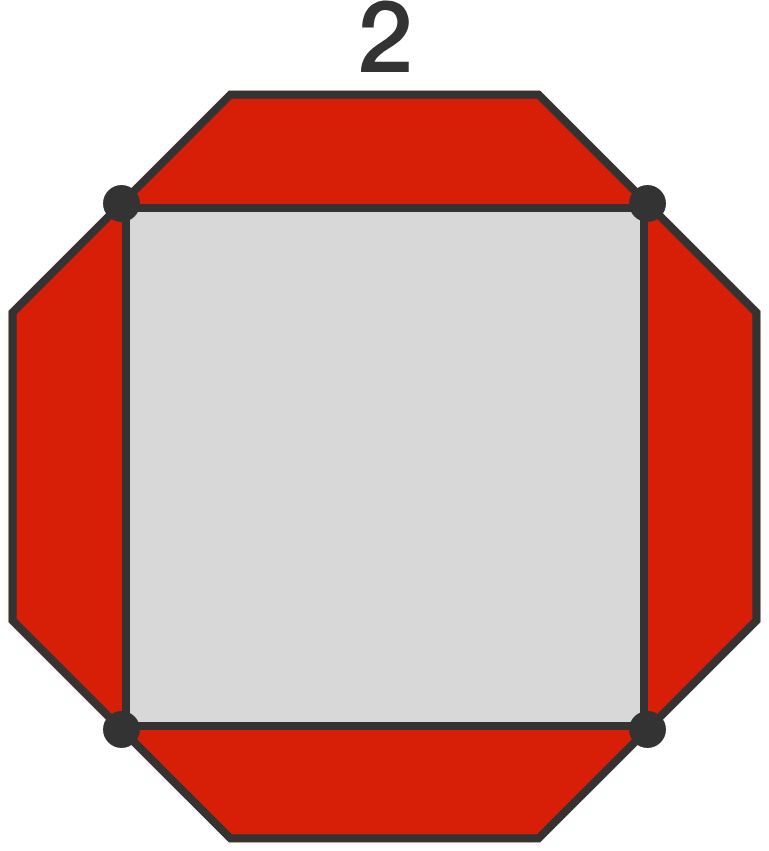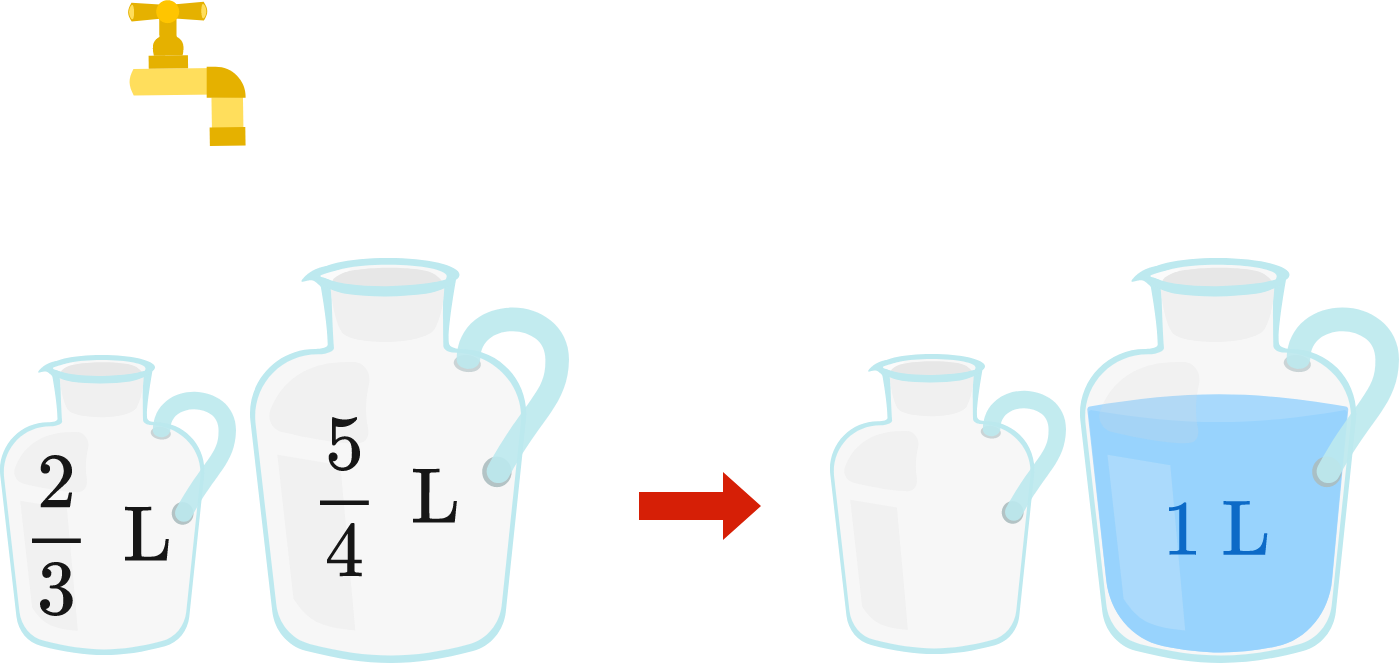# Problems of the Week

Contribute a problem

# 2017-12-25 IntermediateA bunch of bananas of total weight $W$ is hung at one end of a string passing over a perfectly smooth pulley. At the other end, a monkey starts climbing up the rope at a constant acceleration and covers 16 ft in 2 seconds.

If the bananas always remain at rest, find the weight of the monkey.

Assume that $g=32 ~ \text{ft/s}^2.$

In the figure, a force of $40\text{ N}$ is applied on a plank of mass $5 \text{ kg}.$ A solid sphere of mass $3 \text{ kg}$ rolls without slipping on the plank. The pulley is light and connected to a rigid wall. The surface between the sphere and plank is sufficiently rough such that the sphere rolls. The floor is frictionless.

Find the acceleration $\big($in $\text{m/s}^2\big)$ of the plank.Are there any three distinct digits $a, b, c$ such that all six permutations $\overline{abc}, \overline{acb}, \overline{bac}, \overline{bca}, \overline{cab}, \overline{cba}$ are prime numbers?A regular octagon has sides that each measure 2 units long.

A square is inscribed inside, where the vertices of the square touch the regular octagon at midpoints, as shown.

What is the area of the shaded red portion to three decimal places?

I have 2 unmarked empty jugs of capacities $\frac{2}{3}$ liters and $\frac{5}{4}$ liters, as shown below left.

Because they are unmarked, I can only pour water from one jug to another until the initial jug is empty or the other is full. The unwanted water will be wasted into a sink, for there are no other containers.

After some pouring/filling/wasting, I measure exactly 1 liter of water in the bigger jug with the other empty, as shown.

Using the minimum amount of water to obtain the 1 liter, let $x$ be the number of times an empty jug is filled from the faucet, and let $y$ be the number of times a full jug is emptied into the sink. What is the value of $x+y?$×